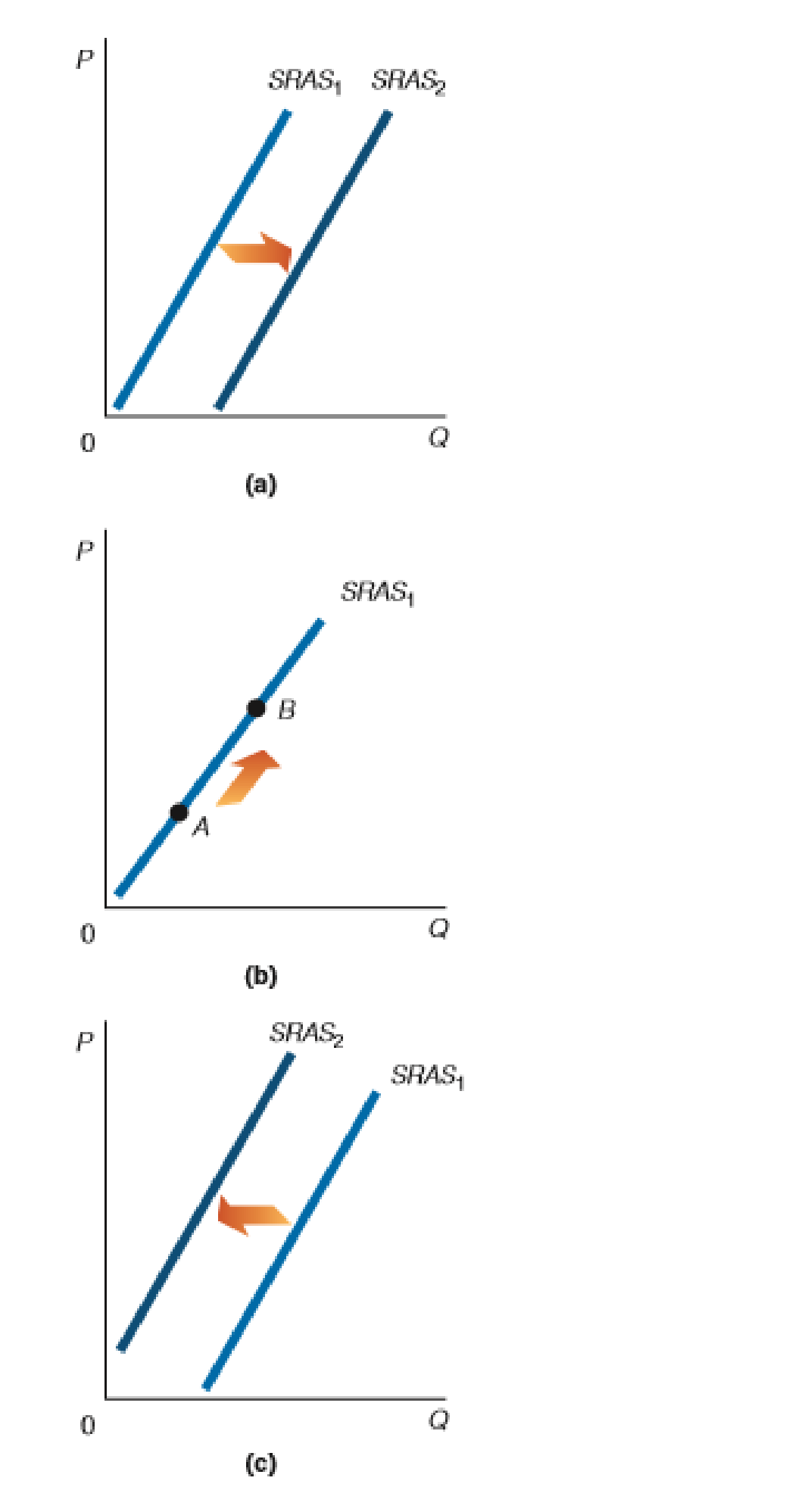Chapter 8, Problem 4WNG

Chapter
Section
Textbook Problem

In the following figure, which part is representative of each of the following? a. A decrease in wage rates b. An increase in the price level c. A beneficial supply shock d. An increase in the price of nonlabor inputs(a)

To determine

Estimate the decrease in wage rates.

Explanation

In an economy, decrease in wage rate causes an increase in the short-run aggregate supply in the market. Thus, increase in the short-run aggregate supply leads to decrease in the price level and increase in the real GDP.

(b)

To determine

Explain an increase in the price level.

(c)

To determine

Explain a beneficial supply shock.

(d)

To determine

Explain an increase in the price of non-labor input.

Still sussing out bartleby?

Check out a sample textbook solution.

See a sample solution

The Solution to Your Study Problems

Bartleby provides explanations to thousands of textbook problems written by our experts, many with advanced degrees!

Get Started